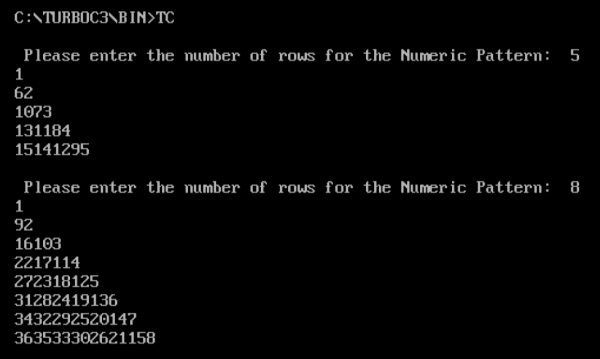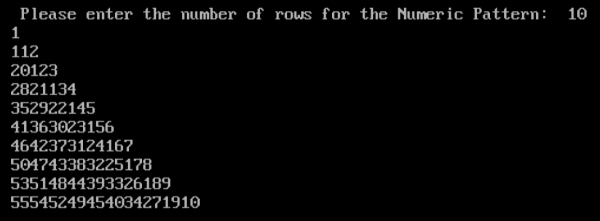# Program to print numeric pattern in C

## Program Description

Print the numeric pattern by accepting the number of rows from the user.

Input: 5 Rows

1
6 2
10 7 3
13 11 8 4
15 14 12 9 5

## Algorithm

Print the pattern from the end of each Row
Complete the last column of each Row
Start from the Second Last Column of the second row
Repeat till the number of rows specified by the User.

## Example

/*Program to print Numeric Pattern */
#include<stdio.h>
int main()
{
int k, l, m, count=1;
int rows;
clrscr();
printf(" Please enter the number of rows for the Numeric Pattern: ");
scanf("%d",&rows);
for (k = 1; k <= rows; k++) {
m = count;
for (l = 1; l <= k; l++) {
printf("%d",m);
m = m - (rows + l - k);
}
printf("");
count = count + 1 + rows - k;
}
getch();
return 0;
}

## Output